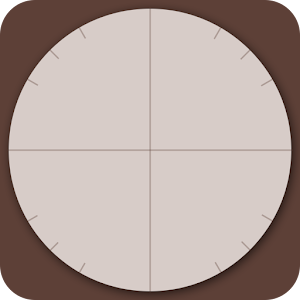## Unit Circle (Interactive Math) helps you practice Grade HS Math - Lumos Educational App Store3.51
Price -NA
\$A

#### DESCRIPTION:

In High School mathematics students will take quizzes and test involving the unit circle. For many it is hard to understand and memorize. This app is designed to help you understand more of the Unit Circle at any math level. Use it to help determine the radius of a circle, sine, cosine, tangent, or for use during a unit circle quiz. ---------------------------------------------------- So What is the Unit Circle? The Unit Circle in mathematics is a circle with a radius of one, with the origin located at (0,0). The Unit Circle is usually used near the end of the High School math curri

#### OVERVIEW:

Unit Circle (Interactive Math) is a free educational mobile app By Jase Owens.It helps students in grades HS practice the following standards HSG.C.A.1,HSG.C.A.2,HSG.C.A.3.

This page not only allows students and teachers download Unit Circle (Interactive Math) but also find engaging Sample Questions, Videos, Pins, Worksheets, Books related to the following topics.

1. HSG.C.A.1 : Prove that all circles are similar.

2. HSG.C.A.2 : Identify and describe relationships among inscribed angles, radii, and chords. Include the relationship between central, inscribed, and circumscribed angles; inscribed angles on a diameter are right angles; the radius of a circle is perpendicular to the tangent where the radius intersects the circle.

3. HSG.C.A.3 : Construct the inscribed and circumscribed circles of a triangle, and prove properties of angles for a quadrilateral inscribed in a circle..

HS

#### STANDARDS:

HSG.C.A.1
HSG.C.A.2
HSG.C.A.3
HSG.C.A.4
HSG.C.B.5

Developer: Jase Owens

Software Version: 2.0.0

Category: Education

### RELATED APPSEdSearch WebSearch